Courses

# Chapter - 2 Gaseous State Class 12 Notes | EduRev

## Class 12 : Chapter - 2 Gaseous State Class 12 Notes | EduRev

The document Chapter - 2 Gaseous State Class 12 Notes | EduRev is a part of the Class 12 Course Chemistry Class 12.
All you need of Class 12 at this link: Class 12

Temperature Scale :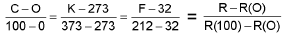where R = Temp, on unknown scale.

Boyle’s law and measurement of pressure :

At constant temperature,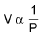P1V1 = P2V2

Charles law :

At constant pressure, V α T or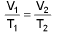Gay-lussac’s law :

At constant volume, P α T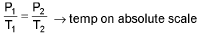Ideal gas Equation :

PV = nRT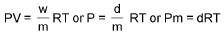Daltons law of partial pressure :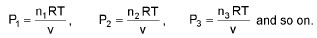Total pressure = P1 + P2 + P3 + ....

Partial pressure = mole fraction X Total pressure.

Amagat’s law of partial volume :

V = V1 + V2 + V3 + ....

Average molecular mass of gaseous mixture :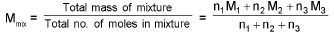Graham’s Law:

Rate of diffusion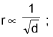d = density of gas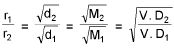Kinetic Theory of Gases :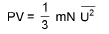Kinetic equation of gases

Average K.E. for one mole =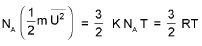• Root mean suqare speed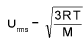molar mass must be in kg/mole.
• Average speed
• Uav = U1 + U2 + U3 + .... UN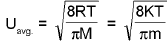K is Boltzmman constant
• Most probable speed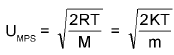Vander wall’s equation :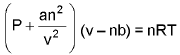• Critical constants:
Vc = 3b,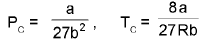Vander wall equation in virial form :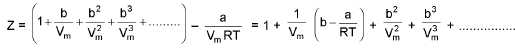Reduced Equation of state :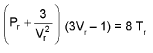Offer running on EduRev: Apply code STAYHOME200 to get INR 200 off on our premium plan EduRev Infinity!

## Chemistry Class 12

121 videos|250 docs|199 tests

,

,

,

,

,

,

,

,

,

,

,

,

,

,

,

,

,

,

,

,

,

;